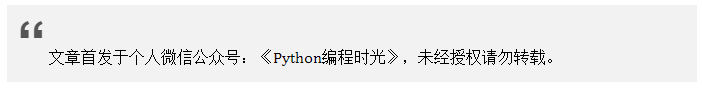# 5.1 图解九大经典排序算法¶## 5.1.1 快速排序¶

def quick_sort(array, reverse=False):
if len(array) > 0:
pivot = array
# 这里使用列表生成式，是为了代码可读性。
# 这里其实应该使用的是for循环，不然每递归一次就多循环了一次。
less = [x for x in array if x < pivot]
greater = [x for x in array if x > pivot]
# 正序
if not reverse:
return  quick_sort(less, reverse) + [pivot] + quick_sort(greater, reverse)
# 倒序
else:
return quick_sort(greater, reverse) + [pivot] + quick_sort(less, reverse)
return []


## 5.1.2 冒泡排序¶

def bubble_sort(array, reverse=False):
length = len(array)
# 循环length次，每一次都选出一个最大/小值，浮到最后。
for i in range(length):
end = length-i
for k in range(end):
# 正序
if not reverse:
if k+1 < end and array[k] > array[k+1]:
array[k+1],array[k] = array[k],array[k+1]
# 倒序
else:
if k+1 < end and array[k] < array[k+1]:
array[k+1],array[k] = array[k],array[k+1]
return array


## 5.1.3 选择排序¶

def select_sort(array, reverse=False):
length = len(array)
for x in range(length):
# 每次循环里，最大或者最小值的索引：边缘索引
edge_index = 0
for y in range(length-x):
# 正序
if not reverse:
if array[y] > array[edge_index]:
edge_index = y
# 倒序
else:
if array[y] < array[edge_index]:
edge_index = y
array[length-x-1],array[edge_index] = array[edge_index],array[length-x-1]
return array


## 5.1.4 插入排序¶

def insert_sort(array,reverse=False):
length = len(array)
for index in range(1,length):
# 当前要比较的值
current_value = array[index]
position = index
# 正序
if not reverse:
while position>0 and array[position-1]>current_value:
array[position] = array[position-1]
position -= 1
array[position] = current_value
# 倒序
else:
while position>0 and array[position-1]<current_value:
array[position] = array[position-1]
position -= 1
array[position] = current_value
return array


def insert_sort(array):
for i in range(len(array)):
for j in range(i):
if array[i] < array[j]:
array.insert(j, array.pop(i))
break
return array


## 5.1.5 希尔排序¶def shell_sort(array, reverse=False):
length = len(array)
# 长度的一半，>> 是位运算，相当于是"length//2"
group_num = length >> 1
while group_num > 0:
for i in range(group_num, length):
current_index_value = array[i]
# k的作用：用于当前索引往前移动n个group_num的索引
k = i
# while循环把同一组的最小值移到最前
# 正序
if not reverse:
while k >= group_num and array[k-group_num] > current_index_value:
array[k] = array[k-group_num]
# 索引往前移动
k -= group_num
# 倒序
else:
while k >= group_num and array[k-group_num] < current_index_value:
array[k] = array[k-group_num]
# 索引往前移动
k -= group_num
array[k] = current_index_value

group_num >>= 1
return array


## 5.1.6 堆排序¶

# 建立最大堆
def build_max_heap(arr, start, end):
root = start
while True:
# 从root开始对最大堆调整
child = 2 * root + 1
# 避免和已排过的数再比较
if child > end:
break

# 找出两个child中较大的一个
if child + 1 <= end and arr[child] < arr[child + 1]:
child += 1

if arr[root] < arr[child]:
# 最大堆小于较大的child, 交换顺序
arr[root], arr[child] = arr[child], arr[root]

# 正在调整的节点设置为root
root = child
else:
# 无需调整的时候, 退出
break

def heap_sort(arr):
# 从最后一个有子节点的节点开始，调整最大堆
first = len(arr) // 2 - 1
for start in range(first, -1, -1):
build_max_heap(arr, start, len(arr) - 1)

# 将最大的放到堆的最后一个, 堆-1, 继续调整排序
for end in range(len(arr) -1, 0, -1):
arr, arr[end] = arr[end], arr
build_max_heap(arr, 0, end - 1)
return arr


def build_min_heap(arr, start, end):
root = start
while True:
# 从root开始对最小堆调整
child = 2 * root + 1
# 避免和已排过的数再比较
if child > end:
break

# 找出两个child中较小的一个
if child + 1 <= end and arr[child] > arr[child + 1]:
child += 1

if arr[root] > arr[child]:
# 最大堆小于较小的child, 交换顺序
arr[root], arr[child] = arr[child], arr[root]

# 正在调整的节点设置为root
root = child
else:
# 无需调整的时候, 退出
break


## 5.1.7 归并排序¶

# 分：分割最小化数组
def merge_sort(seq):
if len(seq) <= 1:
return seq
mid = int(len(seq) / 2)
left = merge_sort(seq[:mid])
right = merge_sort(seq[mid:])
return merge(left, right)

# 治：合并两有序数组
def merge(left, right):
result = []
i, j = 0, 0
while i < len(left) and j < len(right):
if left[i] <= right[j]:
result.append(left[i])
i += 1
else:
result.append(right[j])
j += 1
result += left[i:]
result += right[j:]
return result


## 5.1.8 桶排序¶

def bucket_sort(array, reverse=False):
_max = max(array)
_min = min(array)
# 桶的个数
buckets =  * (_max - _min + 1)
# 在桶里记录每个数出现的频率
for i in array:
buckets[i-_min] += 1

sorted_list = []
for index, item in enumerate(buckets):
if item != 0:
# 重复的数值要存储多次
for x in range(item):
sorted_list.append(index+_min)
# 正序
if not reverse:
return sorted_list
# 倒序
else:
return sorted_list[::-1]


## 5.1.9基数排序¶

def radix_sort(array, reverse=False):
# 最大的数有几位，决定了要几轮排序
d = len(str(max(array)))
for k in range(d):
# 因为每一位数字都是0~9，故建立10个桶
buckets=[[] for i in range(10)]
for i in array:
# 举例：132//10=13，13%10=3
buckets[i//(10**k)%10].append(i)
array=[item for items in buckets for item in items]

if not reverse:
return array
return array[::-1]


## 附录：参考文章¶